## 5.419. visible

Origin

Extension of accessibility parameter of $\mathrm{𝚍𝚒𝚏𝚏𝚗}$.

Constraint

$\mathrm{𝚟𝚒𝚜𝚒𝚋𝚕𝚎}\left(𝙺,\mathrm{𝙳𝙸𝙼𝚂},\mathrm{𝙵𝚁𝙾𝙼},\mathrm{𝙾𝙱𝙹𝙴𝙲𝚃𝚂},\mathrm{𝚂𝙱𝙾𝚇𝙴𝚂}\right)$

Types
 $\mathrm{𝚅𝙰𝚁𝙸𝙰𝙱𝙻𝙴𝚂}$ $\mathrm{𝚌𝚘𝚕𝚕𝚎𝚌𝚝𝚒𝚘𝚗}\left(𝚟-\mathrm{𝚍𝚟𝚊𝚛}\right)$ $\mathrm{𝙸𝙽𝚃𝙴𝙶𝙴𝚁𝚂}$ $\mathrm{𝚌𝚘𝚕𝚕𝚎𝚌𝚝𝚒𝚘𝚗}\left(𝚟-\mathrm{𝚒𝚗𝚝}\right)$ $\mathrm{𝙿𝙾𝚂𝙸𝚃𝙸𝚅𝙴𝚂}$ $\mathrm{𝚌𝚘𝚕𝚕𝚎𝚌𝚝𝚒𝚘𝚗}\left(𝚟-\mathrm{𝚒𝚗𝚝}\right)$ $\mathrm{𝙳𝙸𝙼𝙳𝙸𝚁}$ $\mathrm{𝚌𝚘𝚕𝚕𝚎𝚌𝚝𝚒𝚘𝚗}\left(\mathrm{𝚍𝚒𝚖}-\mathrm{𝚒𝚗𝚝},\mathrm{𝚍𝚒𝚛}-\mathrm{𝚒𝚗𝚝}\right)$
Arguments
 $𝙺$ $\mathrm{𝚒𝚗𝚝}$ $\mathrm{𝙳𝙸𝙼𝚂}$ $\mathrm{𝚜𝚒𝚗𝚝}$ $\mathrm{𝙵𝚁𝙾𝙼}$ $\mathrm{𝙳𝙸𝙼𝙳𝙸𝚁}$ $\mathrm{𝙾𝙱𝙹𝙴𝙲𝚃𝚂}$ $\mathrm{𝚌𝚘𝚕𝚕𝚎𝚌𝚝𝚒𝚘𝚗}\left(\begin{array}{c}\mathrm{𝚘𝚒𝚍}-\mathrm{𝚒𝚗𝚝},\hfill \\ \mathrm{𝚜𝚒𝚍}-\mathrm{𝚍𝚟𝚊𝚛},\hfill \\ 𝚡-\mathrm{𝚅𝙰𝚁𝙸𝙰𝙱𝙻𝙴𝚂},\hfill \\ \mathrm{𝚜𝚝𝚊𝚛𝚝}-\mathrm{𝚍𝚟𝚊𝚛},\hfill \\ \mathrm{𝚍𝚞𝚛𝚊𝚝𝚒𝚘𝚗}-\mathrm{𝚍𝚟𝚊𝚛},\hfill \\ \mathrm{𝚎𝚗𝚍}-\mathrm{𝚍𝚟𝚊𝚛}\hfill \end{array}\right)$ $\mathrm{𝚂𝙱𝙾𝚇𝙴𝚂}$ $\mathrm{𝚌𝚘𝚕𝚕𝚎𝚌𝚝𝚒𝚘𝚗}\left(\begin{array}{c}\mathrm{𝚜𝚒𝚍}-\mathrm{𝚒𝚗𝚝},\hfill \\ 𝚝-\mathrm{𝙸𝙽𝚃𝙴𝙶𝙴𝚁𝚂},\hfill \\ 𝚕-\mathrm{𝙿𝙾𝚂𝙸𝚃𝙸𝚅𝙴𝚂},\hfill \\ 𝚏-\mathrm{𝙳𝙸𝙼𝙳𝙸𝚁}\hfill \end{array}\right)$
Restrictions
 $|\mathrm{𝚅𝙰𝚁𝙸𝙰𝙱𝙻𝙴𝚂}|\ge 1$ $|\mathrm{𝙸𝙽𝚃𝙴𝙶𝙴𝚁𝚂}|\ge 1$ $|\mathrm{𝙿𝙾𝚂𝙸𝚃𝙸𝚅𝙴𝚂}|\ge 1$ $\mathrm{𝚛𝚎𝚚𝚞𝚒𝚛𝚎𝚍}$$\left(\mathrm{𝚅𝙰𝚁𝙸𝙰𝙱𝙻𝙴𝚂},𝚟\right)$ $|\mathrm{𝚅𝙰𝚁𝙸𝙰𝙱𝙻𝙴𝚂}|=𝙺$ $\mathrm{𝚛𝚎𝚚𝚞𝚒𝚛𝚎𝚍}$$\left(\mathrm{𝙸𝙽𝚃𝙴𝙶𝙴𝚁𝚂},𝚟\right)$ $|\mathrm{𝙸𝙽𝚃𝙴𝙶𝙴𝚁𝚂}|=𝙺$ $\mathrm{𝚛𝚎𝚚𝚞𝚒𝚛𝚎𝚍}$$\left(\mathrm{𝙿𝙾𝚂𝙸𝚃𝙸𝚅𝙴𝚂},𝚟\right)$ $|\mathrm{𝙿𝙾𝚂𝙸𝚃𝙸𝚅𝙴𝚂}|=𝙺$ $\mathrm{𝙿𝙾𝚂𝙸𝚃𝙸𝚅𝙴𝚂}.𝚟>0$ $\mathrm{𝚛𝚎𝚚𝚞𝚒𝚛𝚎𝚍}$$\left(\mathrm{𝙳𝙸𝙼𝙳𝙸𝚁},\left[\mathrm{𝚍𝚒𝚖},\mathrm{𝚍𝚒𝚛}\right]\right)$ $|\mathrm{𝙳𝙸𝙼𝙳𝙸𝚁}|>0$ $|\mathrm{𝙳𝙸𝙼𝙳𝙸𝚁}|\le 𝙺+𝙺$ $\mathrm{𝚍𝚒𝚜𝚝𝚒𝚗𝚌𝚝}$$\left(\mathrm{𝙳𝙸𝙼𝙳𝙸𝚁},\left[\right]\right)$ $\mathrm{𝙳𝙸𝙼𝙳𝙸𝚁}.\mathrm{𝚍𝚒𝚖}\ge 0$ $\mathrm{𝙳𝙸𝙼𝙳𝙸𝚁}.\mathrm{𝚍𝚒𝚖}<𝙺$ $\mathrm{𝙳𝙸𝙼𝙳𝙸𝚁}.\mathrm{𝚍𝚒𝚛}\ge 0$ $\mathrm{𝙳𝙸𝙼𝙳𝙸𝚁}.\mathrm{𝚍𝚒𝚛}\le 1$ $𝙺\ge 0$ $\mathrm{𝙳𝙸𝙼𝚂}\ge 0$ $\mathrm{𝙳𝙸𝙼𝚂}<𝙺$ $\mathrm{𝚍𝚒𝚜𝚝𝚒𝚗𝚌𝚝}$$\left(\mathrm{𝙾𝙱𝙹𝙴𝙲𝚃𝚂},\mathrm{𝚘𝚒𝚍}\right)$ $\mathrm{𝚛𝚎𝚚𝚞𝚒𝚛𝚎𝚍}$$\left(\mathrm{𝙾𝙱𝙹𝙴𝙲𝚃𝚂},\left[\mathrm{𝚘𝚒𝚍},\mathrm{𝚜𝚒𝚍},𝚡\right]\right)$ $\mathrm{𝚛𝚎𝚚𝚞𝚒𝚛𝚎}_\mathrm{𝚊𝚝}_\mathrm{𝚕𝚎𝚊𝚜𝚝}$$\left(2,\mathrm{𝙾𝙱𝙹𝙴𝙲𝚃𝚂},\left[\mathrm{𝚜𝚝𝚊𝚛𝚝},\mathrm{𝚍𝚞𝚛𝚊𝚝𝚒𝚘𝚗},\mathrm{𝚎𝚗𝚍}\right]\right)$ $\mathrm{𝙾𝙱𝙹𝙴𝙲𝚃𝚂}.\mathrm{𝚘𝚒𝚍}\ge 1$ $\mathrm{𝙾𝙱𝙹𝙴𝙲𝚃𝚂}.\mathrm{𝚘𝚒𝚍}\le |\mathrm{𝙾𝙱𝙹𝙴𝙲𝚃𝚂}|$ $\mathrm{𝙾𝙱𝙹𝙴𝙲𝚃𝚂}.\mathrm{𝚜𝚒𝚍}\ge 1$ $\mathrm{𝙾𝙱𝙹𝙴𝙲𝚃𝚂}.\mathrm{𝚜𝚒𝚍}\le |\mathrm{𝚂𝙱𝙾𝚇𝙴𝚂}|$ $\mathrm{𝙾𝙱𝙹𝙴𝙲𝚃𝚂}.\mathrm{𝚍𝚞𝚛𝚊𝚝𝚒𝚘𝚗}\ge 0$ $|\mathrm{𝚂𝙱𝙾𝚇𝙴𝚂}|\ge 1$ $\mathrm{𝚛𝚎𝚚𝚞𝚒𝚛𝚎𝚍}$$\left(\mathrm{𝚂𝙱𝙾𝚇𝙴𝚂},\left[\mathrm{𝚜𝚒𝚍},𝚝,𝚕\right]\right)$ $\mathrm{𝚂𝙱𝙾𝚇𝙴𝚂}.\mathrm{𝚜𝚒𝚍}\ge 1$ $\mathrm{𝚂𝙱𝙾𝚇𝙴𝚂}.\mathrm{𝚜𝚒𝚍}\le |\mathrm{𝚂𝙱𝙾𝚇𝙴𝚂}|$ $\mathrm{𝚍𝚘}_\mathrm{𝚗𝚘𝚝}_\mathrm{𝚘𝚟𝚎𝚛𝚕𝚊𝚙}\left(\mathrm{𝚂𝙱𝙾𝚇𝙴𝚂}\right)$
Purpose

Holds if and only if:

1. The difference between the end in time and the start in time of each object is equal to its duration in time.

2. Given a collection of potential observations places $\mathrm{𝙵𝚁𝙾𝙼}$, where each observation place is specified by a dimension (i.e., an integer between 0 and $k-1$) and by a direction (i.e., an integer between 0 and 1), and given for each shifted box of $\mathrm{𝚂𝙱𝙾𝚇𝙴𝚂}$ a set of visible faces, enforce that at least one visible face of each shifted box associated with an object $o\in \mathrm{𝙾𝙱𝙹𝙴𝙲𝚃𝚂}$ should be entirely visible from at least one observation place of $\mathrm{𝙵𝚁𝙾𝙼}$ at time $o.\mathrm{𝚜𝚝𝚊𝚛𝚝}$ as well as at time $o.\mathrm{𝚎𝚗𝚍}-1$. This notion is defined in a more formal way in the Remark slot.

Example
 $\left(\begin{array}{c}2,\left\{0,1\right\},\hfill \\ 〈\mathrm{𝚍𝚒𝚖}-0\mathrm{𝚍𝚒𝚛}-1〉,\hfill \\ 〈\begin{array}{cccccc}\mathrm{𝚘𝚒𝚍}-1\hfill & \mathrm{𝚜𝚒𝚍}-1\hfill & 𝚡-〈1,2〉\hfill & \mathrm{𝚜𝚝𝚊𝚛𝚝}-8\hfill & \mathrm{𝚍𝚞𝚛𝚊𝚝𝚒𝚘𝚗}-8\hfill & \mathrm{𝚎𝚗𝚍}-16,\hfill \\ \mathrm{𝚘𝚒𝚍}-2\hfill & \mathrm{𝚜𝚒𝚍}-2\hfill & 𝚡-〈4,2〉\hfill & \mathrm{𝚜𝚝𝚊𝚛𝚝}-1\hfill & \mathrm{𝚍𝚞𝚛𝚊𝚝𝚒𝚘𝚗}-15\hfill & \mathrm{𝚎𝚗𝚍}-16\hfill \end{array}〉,\hfill \\ 〈\begin{array}{cccc}\mathrm{𝚜𝚒𝚍}-1\hfill & 𝚝-〈0,0〉\hfill & 𝚕-〈1,2〉\hfill & 𝚏-〈\mathrm{𝚍𝚒𝚖}-0\mathrm{𝚍𝚒𝚛}-1〉,\hfill \\ \mathrm{𝚜𝚒𝚍}-2\hfill & 𝚝-〈0,0〉\hfill & 𝚕-〈2,3〉\hfill & 𝚏-〈\mathrm{𝚍𝚒𝚖}-0\mathrm{𝚍𝚒𝚛}-1〉\hfill \end{array}〉\hfill \end{array}\right)$ $\left(\begin{array}{c}2,\left\{0,1\right\},\hfill \\ 〈\mathrm{𝚍𝚒𝚖}-0\mathrm{𝚍𝚒𝚛}-1〉,\hfill \\ 〈\begin{array}{cccccc}\mathrm{𝚘𝚒𝚍}-1\hfill & \mathrm{𝚜𝚒𝚍}-1\hfill & 𝚡-〈1,2〉\hfill & \mathrm{𝚜𝚝𝚊𝚛𝚝}-1\hfill & \mathrm{𝚍𝚞𝚛𝚊𝚝𝚒𝚘𝚗}-8\hfill & \mathrm{𝚎𝚗𝚍}-9,\hfill \\ \mathrm{𝚘𝚒𝚍}-2\hfill & \mathrm{𝚜𝚒𝚍}-2\hfill & 𝚡-〈4,2〉\hfill & \mathrm{𝚜𝚝𝚊𝚛𝚝}-1\hfill & \mathrm{𝚍𝚞𝚛𝚊𝚝𝚒𝚘𝚗}-15\hfill & \mathrm{𝚎𝚗𝚍}-16\hfill \end{array}〉,\hfill \\ 〈\begin{array}{cccc}\mathrm{𝚜𝚒𝚍}-1\hfill & 𝚝-〈0,0〉\hfill & 𝚕-〈1,2〉\hfill & 𝚏-〈\mathrm{𝚍𝚒𝚖}-0\mathrm{𝚍𝚒𝚛}-1〉,\hfill \\ \mathrm{𝚜𝚒𝚍}-2\hfill & 𝚝-〈0,0〉\hfill & 𝚕-〈2,3〉\hfill & 𝚏-〈\mathrm{𝚍𝚒𝚖}-0\mathrm{𝚍𝚒𝚛}-1〉\hfill \end{array}〉\hfill \end{array}\right)$ $\left(\begin{array}{c}2,\left\{0,1\right\},\hfill \\ 〈\mathrm{𝚍𝚒𝚖}-0\mathrm{𝚍𝚒𝚛}-1〉,\hfill \\ 〈\begin{array}{cccccc}\mathrm{𝚘𝚒𝚍}-1\hfill & \mathrm{𝚜𝚒𝚍}-1\hfill & 𝚡-〈1,1〉\hfill & \mathrm{𝚜𝚝𝚊𝚛𝚝}-1\hfill & \mathrm{𝚍𝚞𝚛𝚊𝚝𝚒𝚘𝚗}-15\hfill & \mathrm{𝚎𝚗𝚍}-16,\hfill \\ \mathrm{𝚘𝚒𝚍}-2\hfill & \mathrm{𝚜𝚒𝚍}-2\hfill & 𝚡-〈2,2〉\hfill & \mathrm{𝚜𝚝𝚊𝚛𝚝}-6\hfill & \mathrm{𝚍𝚞𝚛𝚊𝚝𝚒𝚘𝚗}-6\hfill & \mathrm{𝚎𝚗𝚍}-12\hfill \end{array}〉,\hfill \\ 〈\begin{array}{cccc}\mathrm{𝚜𝚒𝚍}-1\hfill & 𝚝-〈0,0〉\hfill & 𝚕-〈1,2〉\hfill & 𝚏-〈\mathrm{𝚍𝚒𝚖}-0\mathrm{𝚍𝚒𝚛}-1〉,\hfill \\ \mathrm{𝚜𝚒𝚍}-2\hfill & 𝚝-〈0,0〉\hfill & 𝚕-〈2,3〉\hfill & 𝚏-〈\mathrm{𝚍𝚒𝚖}-0\mathrm{𝚍𝚒𝚛}-1〉\hfill \end{array}〉\hfill \end{array}\right)$ $\left(\begin{array}{c}2,\left\{0,1\right\},\hfill \\ 〈\mathrm{𝚍𝚒𝚖}-0\mathrm{𝚍𝚒𝚛}-1〉,\hfill \\ 〈\begin{array}{cccccc}\mathrm{𝚘𝚒𝚍}-1\hfill & \mathrm{𝚜𝚒𝚍}-1\hfill & 𝚡-〈4,1〉\hfill & \mathrm{𝚜𝚝𝚊𝚛𝚝}-1\hfill & \mathrm{𝚍𝚞𝚛𝚊𝚝𝚒𝚘𝚗}-8\hfill & \mathrm{𝚎𝚗𝚍}-9,\hfill \\ \mathrm{𝚘𝚒𝚍}-2\hfill & \mathrm{𝚜𝚒𝚍}-2\hfill & 𝚡-〈1,2〉\hfill & \mathrm{𝚜𝚝𝚊𝚛𝚝}-1\hfill & \mathrm{𝚍𝚞𝚛𝚊𝚝𝚒𝚘𝚗}-15\hfill & \mathrm{𝚎𝚗𝚍}-16\hfill \end{array}〉,\hfill \\ 〈\begin{array}{cccc}\mathrm{𝚜𝚒𝚍}-1\hfill & 𝚝-〈0,0〉\hfill & 𝚕-〈1,2〉\hfill & 𝚏-〈\mathrm{𝚍𝚒𝚖}-0\mathrm{𝚍𝚒𝚛}-1〉,\hfill \\ \mathrm{𝚜𝚒𝚍}-2\hfill & 𝚝-〈0,0〉\hfill & 𝚕-〈2,3〉\hfill & 𝚏-〈\mathrm{𝚍𝚒𝚖}-0\mathrm{𝚍𝚒𝚛}-1〉\hfill \end{array}〉\hfill \end{array}\right)$ $\left(\begin{array}{c}2,\left\{0\right\},\hfill \\ 〈\mathrm{𝚍𝚒𝚖}-0\mathrm{𝚍𝚒𝚛}-1〉,\hfill \\ 〈\begin{array}{cccccc}\mathrm{𝚘𝚒𝚍}-1\hfill & \mathrm{𝚜𝚒𝚍}-1\hfill & 𝚡-〈2,1〉\hfill & \mathrm{𝚜𝚝𝚊𝚛𝚝}-1\hfill & \mathrm{𝚍𝚞𝚛𝚊𝚝𝚒𝚘𝚗}-8\hfill & \mathrm{𝚎𝚗𝚍}-9,\hfill \\ \mathrm{𝚘𝚒𝚍}-2\hfill & \mathrm{𝚜𝚒𝚍}-2\hfill & 𝚡-〈4,3〉\hfill & \mathrm{𝚜𝚝𝚊𝚛𝚝}-1\hfill & \mathrm{𝚍𝚞𝚛𝚊𝚝𝚒𝚘𝚗}-15\hfill & \mathrm{𝚎𝚗𝚍}-16\hfill \end{array}〉,\hfill \\ 〈\begin{array}{cccc}\mathrm{𝚜𝚒𝚍}-1\hfill & 𝚝-〈0,0〉\hfill & 𝚕-〈1,2〉\hfill & 𝚏-〈\mathrm{𝚍𝚒𝚖}-0\mathrm{𝚍𝚒𝚛}-1〉,\hfill \\ \mathrm{𝚜𝚒𝚍}-2\hfill & 𝚝-〈0,0〉\hfill & 𝚕-〈2,2〉\hfill & 𝚏-〈\mathrm{𝚍𝚒𝚖}-0\mathrm{𝚍𝚒𝚛}-1〉\hfill \end{array}〉\hfill \end{array}\right)$

The five previous examples correspond respectively to parts (I), (II) of Figure 5.419.2, to parts (III) and (IV) of Figure 5.419.3, and to Figure 5.419.4. Before introducing these five examples Figure 5.419.1 first illustrates the notion of observations places and of visible faces.

##### Figure 5.419.1. Entirely visible faces (depicted by a thick line) of rectangles ①, ②, ③, ④, ⑤, ⑥ and ⑦ from the four observation places $〈\mathrm{𝚍𝚒𝚖}=0,\mathrm{𝚍𝚒𝚛}=1〉$, $〈\mathrm{𝚍𝚒𝚖}=0,\mathrm{𝚍𝚒𝚛}=0〉$, $〈\mathrm{𝚍𝚒𝚖}=1,\mathrm{𝚍𝚒𝚛}=1〉$ and $〈\mathrm{𝚍𝚒𝚖}=1,\mathrm{𝚍𝚒𝚛}=0〉$ (depicted by a small triangle)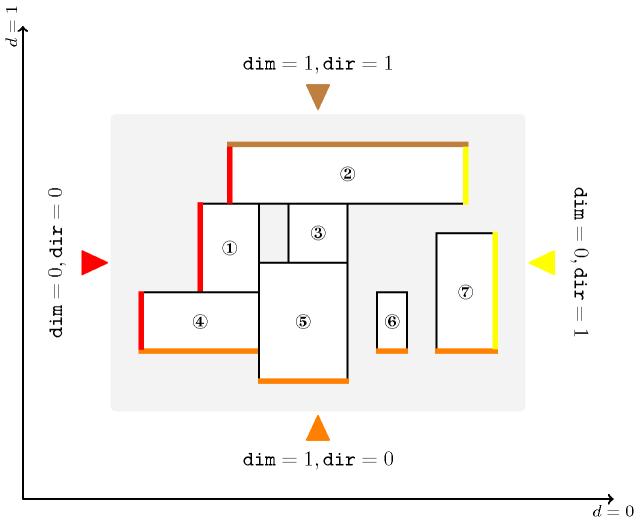We first need to introduce a number of definitions in order to illustrate the notion of visibility.

Definition 1 Consider two distinct objects $o$ and ${o}^{\text{'}}$ of the $\mathrm{𝚟𝚒𝚜𝚒𝚋𝚕𝚎}$ constraint (i.e., $o,{o}^{\text{'}}\in \mathrm{𝑖𝑜𝑏𝑗𝑒𝑐𝑡𝑠}$) as well as an observation place defined by the pair $〈\mathrm{𝚍𝚒𝚖},\mathrm{𝚍𝚒𝚛}〉\in \mathrm{𝙵𝚁𝙾𝙼}$. The object $o$ is masked by the object ${o}^{\text{'}}$ according to the observation place $〈\mathrm{𝚍𝚒𝚖},\mathrm{𝚍𝚒𝚛}〉$ if there exist two shifted boxes $s$ and ${s}^{\text{'}}$ respectively associated with $o$ and ${o}^{\text{'}}$ such that conditions A, B, C, D and E all hold:

• (A) $o.\mathrm{𝚍𝚞𝚛𝚊𝚝𝚒𝚘𝚗}>0\wedge {o}^{\text{'}}.\mathrm{𝚍𝚞𝚛𝚊𝚝𝚒𝚘𝚗}>0\wedge o.\mathrm{𝚎𝚗𝚍}>{o}^{\text{'}}.\mathrm{𝚜𝚝𝚊𝚛𝚝}\wedge {o}^{\text{'}}.\mathrm{𝚎𝚗𝚍}>o.\mathrm{𝚜𝚝𝚊𝚛𝚝}$ (i.e., the time intervals associated with $o$ and ${o}^{\text{'}}$ intersect).

• (B) Discarding dimension $\mathrm{𝚍𝚒𝚖}$, $s$ and ${s}^{\text{'}}$ intersect in all dimensions specified by $\mathrm{𝙳𝙸𝙼𝚂}$ (i.e., objects $o$ and ${o}^{\text{'}}$ are in vis-à-vis).

• (C) If $\mathrm{𝚍𝚒𝚛}=0$

then $o.x\left[\mathrm{𝚍𝚒𝚖}\right]+s.t\left[\mathrm{𝚍𝚒𝚖}\right]\ge {o}^{\text{'}}.x\left[\mathrm{𝚍𝚒𝚖}\right]+{s}^{\text{'}}.t\left[\mathrm{𝚍𝚒𝚖}\right]+{s}^{\text{'}}.l\left[\mathrm{𝚍𝚒𝚖}\right]$

else ${o}^{\text{'}}.x\left[\mathrm{𝚍𝚒𝚖}\right]+{s}^{\text{'}}.t\left[\mathrm{𝚍𝚒𝚖}\right]\ge o.x\left[\mathrm{𝚍𝚒𝚖}\right]+s.t\left[\mathrm{𝚍𝚒𝚖}\right]+s.l\left[\mathrm{𝚍𝚒𝚖}\right]$ (i.e., in dimension $\mathrm{𝚍𝚒𝚖}$, $o$ and ${o}^{\text{'}}$ are ordered in the wrong way according to direction $\mathrm{𝚍𝚒𝚛}$).

• (D) $o.\mathrm{𝚜𝚝𝚊𝚛𝚝}>{o}^{\text{'}}.\mathrm{𝚜𝚝𝚊𝚛𝚝}\vee o.\mathrm{𝚎𝚗𝚍}<{o}^{\text{'}}.\mathrm{𝚎𝚗𝚍}$ (i.e., instants $o.\mathrm{𝚜𝚝𝚊𝚛𝚝}$ or $o.\mathrm{𝚎𝚗𝚍}$ are located within interval $\left[{o}^{\text{'}}.\mathrm{𝚜𝚝𝚊𝚛𝚝},{o}^{\text{'}}.\mathrm{𝚎𝚗𝚍}\right]$; we consider also condition A.).

• (E) The observation place $〈\mathrm{𝚍𝚒𝚖},\mathrm{𝚍𝚒𝚛}〉$ occurs within the list of visible faces associated with the face attribute $𝚏$ of the shifted box $s$ (i.e., the pair $〈\mathrm{𝚍𝚒𝚖},\mathrm{𝚍𝚒𝚛}〉$ is a potentially visible face of $o$).

Definition 2 Consider an object $o$ of the collection $\mathrm{𝙾𝙱𝙹𝙴𝙲𝚃𝚂}$ as well as a possible observation place defined by the pair $〈\mathrm{𝚍𝚒𝚖},\mathrm{𝚍𝚒𝚛}〉$. The object $o$ is masked according to the observation place $〈\mathrm{𝚍𝚒𝚖},\mathrm{𝚍𝚒𝚛}〉$ if and only if at least one of the following conditions holds:

• No shifted box associated with $o$ has the pair $〈\mathrm{𝚍𝚒𝚖},\mathrm{𝚍𝚒𝚛}〉$ as one of its potentially visible face.

• The object $o$ is masked according to the possible observation place $〈\mathrm{𝚍𝚒𝚖},\mathrm{𝚍𝚒𝚛}〉$ by another object ${o}^{\text{'}}$.

Figures 5.419.2, 5.419.3, and  5.419.4 respectively illustrate Definition Definition 1 in the context of an observation place (depicted by a triangle) that is equal to the pair $〈\mathrm{𝚍𝚒𝚖}=0,\mathrm{𝚍𝚒𝚛}=1〉$. Note that, in the context of Figure 5.419.4, as the $\mathrm{𝙳𝙸𝙼𝚂}$ parameter of the $\mathrm{𝚟𝚒𝚜𝚒𝚋𝚕𝚎}$ constraint only mentions dimension 0 (and not dimension 1), one object may be masked by another object even though the two objects do not intersect in any dimension: i.e., only their respective ordering in the dimension $\mathrm{𝚍𝚒𝚖}=0$ as well as their positions in time matter.

##### Figure 5.419.2. Illustration of Definition Definition 1: two examples (I) and (II) where an object $o$ is masked by an object ${o}^{\text{'}}$ according to dimensions $\left\{0,1\right\}$ and to the observation place $〈\mathrm{𝚍𝚒𝚖}=0,\mathrm{𝚍𝚒𝚛}=1〉$ because (A)$o$ and ${o}^{\text{'}}$ intersect in time, (B)$o$ and ${o}^{\text{'}}$ intersect in dimension 1, (C)$o$ and ${o}^{\text{'}}$ are not well ordered according to the observation place, (D) there exists an instant where ${o}^{\text{'}}$ if present (but not $o$) and (E)$〈\mathrm{𝚍𝚒𝚖}=0,\mathrm{𝚍𝚒𝚛}=1〉$ is a potentially visible face of $o$.##### Figure 5.419.3. Illustration of Definition Definition 1: two examples (III) and (IV) where an object $o$ is not masked by an object ${o}^{\text{'}}$ according to the observation place $〈\mathrm{𝚍𝚒𝚖}=0,\mathrm{𝚍𝚒𝚛}=1〉$.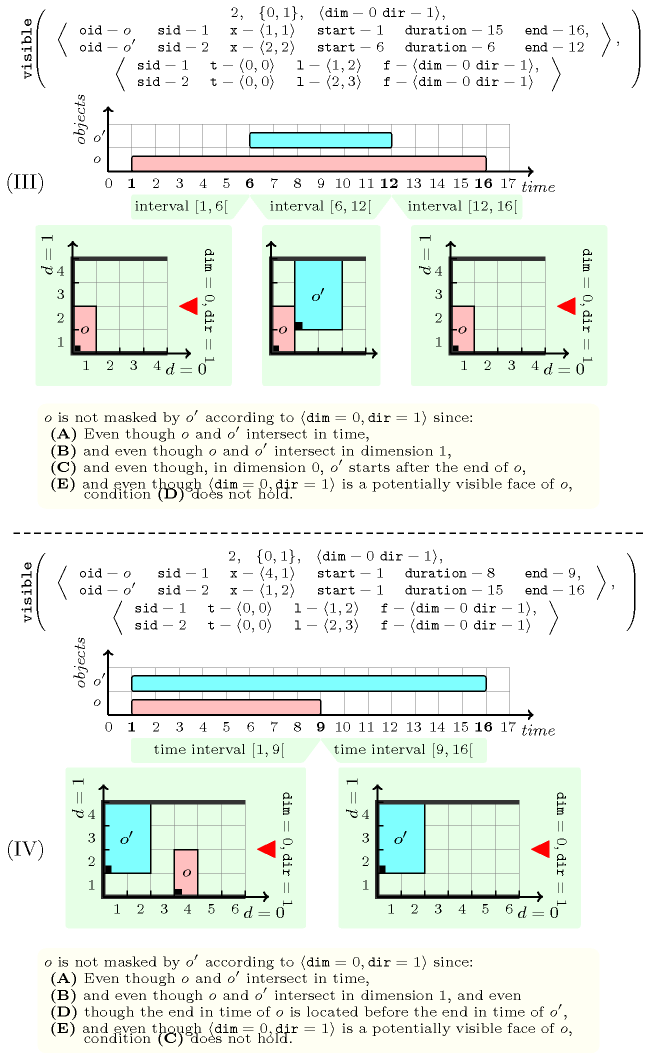##### Figure 5.419.4. Illustration of Definition Definition 1: the case where an object $o$ is masked by an object ${o}^{\text{'}}$ according to dimension 0 and to the observation place $〈\mathrm{𝚍𝚒𝚖}=0,\mathrm{𝚍𝚒𝚛}=1〉$ because: (A)$o$ and ${o}^{\text{'}}$ intersect in time, (C)$o$ and ${o}^{\text{'}}$ are not well ordered according to the observation place and (D) there exists an instant where ${o}^{\text{'}}$ if present (but not $o$) and (E)$〈\mathrm{𝚍𝚒𝚖}=0,\mathrm{𝚍𝚒𝚛}=1〉$ is a potentially visible face of $o$.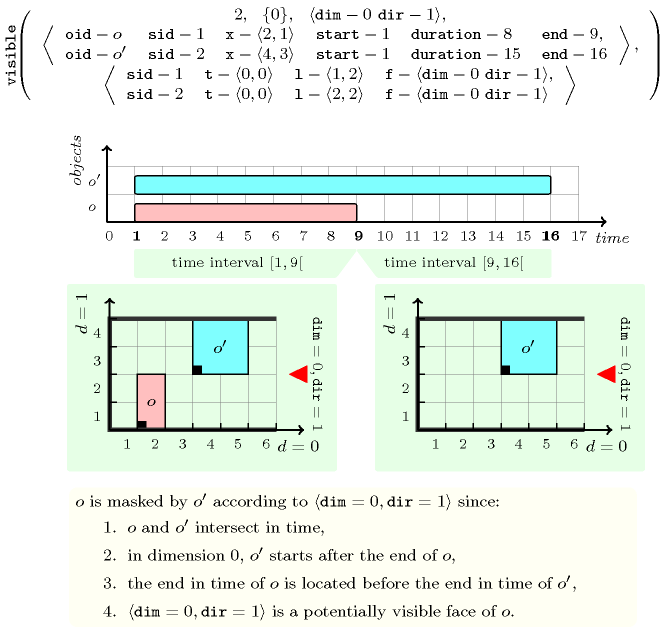Definition 3 Consider an object $o$ of the collection $\mathrm{𝙾𝙱𝙹𝙴𝙲𝚃𝚂}$ as well as a possible observation place defined by the pair $〈\mathrm{𝚍𝚒𝚖},\mathrm{𝚍𝚒𝚛}〉$. The object $o$ is masked according to the observation place $〈\mathrm{𝚍𝚒𝚖},\mathrm{𝚍𝚒𝚛}〉$ if and only if at least one of the following conditions holds:

• No shifted box associated with $o$ has the pair $〈\mathrm{𝚍𝚒𝚖},\mathrm{𝚍𝚒𝚛}〉$ as one of its potentially visible face.

• The object $o$ is masked according to the possible observation place $〈\mathrm{𝚍𝚒𝚖},\mathrm{𝚍𝚒𝚛}〉$ by another object ${o}^{\text{'}}$.

Definition 4 An object of the collection $\mathrm{𝙾𝙱𝙹𝙴𝙲𝚃𝚂}$ constraint is masked according to a set of possible observation places $\mathrm{𝙵𝚁𝙾𝙼}$ if it is masked according to each observation place of $\mathrm{𝙵𝚁𝙾𝙼}$.

We are now in position to define the $\mathrm{𝚟𝚒𝚜𝚒𝚋𝚕𝚎}$ constraint.

Definition 5 Given a $\mathrm{𝚟𝚒𝚜𝚒𝚋𝚕𝚎}$$\left(𝙺,\mathrm{𝙳𝙸𝙼𝚂},\mathrm{𝙵𝚁𝙾𝙼},\mathrm{𝙾𝙱𝙹𝙴𝙲𝚃𝚂},\mathrm{𝚂𝙱𝙾𝚇𝙴𝚂}\right)$ constraint, the $\mathrm{𝚟𝚒𝚜𝚒𝚋𝚕𝚎}$ constraint holds if none of the objects of $\mathrm{𝙾𝙱𝙹𝙴𝙲𝚃𝚂}$ is masked according to the dimensions of $\mathrm{𝙳𝙸𝙼𝚂}$ and to the set of possible observation places defined by $\mathrm{𝙵𝚁𝙾𝙼}$.

Typical
$|\mathrm{𝙾𝙱𝙹𝙴𝙲𝚃𝚂}|>1$
Symmetries
• Items of $\mathrm{𝙾𝙱𝙹𝙴𝙲𝚃𝚂}$ are permutable.

• Items of $\mathrm{𝚂𝙱𝙾𝚇𝙴𝚂}$ are permutable.

Usage

We now give several typical concrete uses of the $\mathrm{𝚟𝚒𝚜𝚒𝚋𝚕𝚎}$ constraint, which all mention the $\mathrm{𝚍𝚒𝚏𝚏𝚜𝚝}$ as well as the $\mathrm{𝚟𝚒𝚜𝚒𝚋𝚕𝚎}$ constraints:

• Figure 5.419.5 corresponds to a ship loading problem where containers are piled within a ship by a crane each time the ship visits a given harbour. In this context we have first to express the fact that a container can only be placed on top of an already placed container and second, that a container can only be taken away if no container is placed on top of it. These two conditions are expressed by a single $\mathrm{𝚟𝚒𝚜𝚒𝚋𝚕𝚎}$ constraint for which the $\mathrm{𝙳𝙸𝙼𝚂}$ parameter mentions all three dimensions of the placement space and the $\mathrm{𝙵𝚁𝙾𝙼}$ parameter mentions the pair $〈\mathrm{𝚍𝚒𝚖}=2,\mathrm{𝚍𝚒𝚛}=1〉$ as its unique observation place. In addition we also use a $\mathrm{𝚍𝚒𝚏𝚏𝚜𝚝}$ constraint for expressing non-overlapping.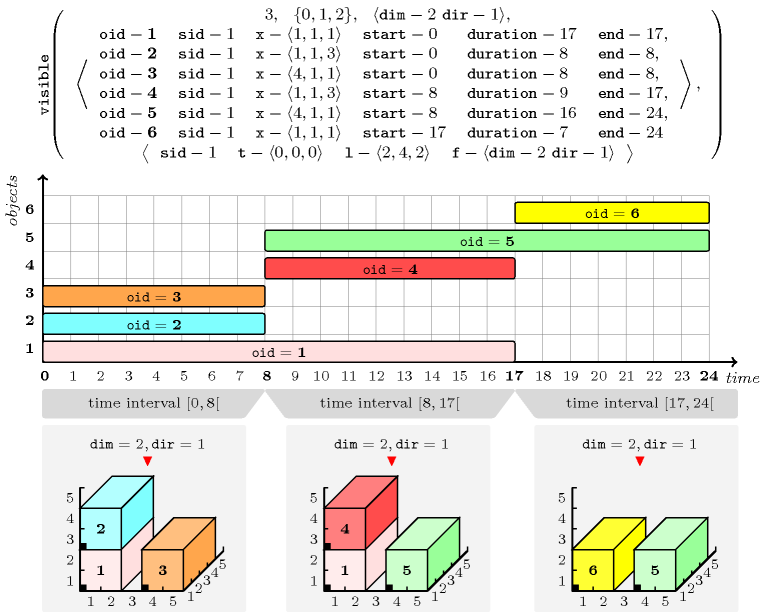• Figure 5.419.6 corresponds to a container loading/unloading problem in the context of a pick-up delivery problem where the loading/unloading takes place with respect to the front door of the container. Beside the $\mathrm{𝚍𝚒𝚏𝚏𝚜𝚝}$ constraint used for expressing non-overlapping, we use two distinct $\mathrm{𝚟𝚒𝚜𝚒𝚋𝚕𝚎}$ constraints:

• The first $\mathrm{𝚟𝚒𝚜𝚒𝚋𝚕𝚎}$ constraint takes care of the location of the front door of the container (each object $o$ has to be loaded/unloaded without moving around any other object, i.e., objects that are in the vis-à-vis of $o$ according to the front door of the container). This is expressed by a single $\mathrm{𝚟𝚒𝚜𝚒𝚋𝚕𝚎}$ constraint for which the $\mathrm{𝙳𝙸𝙼𝚂}$ parameter mentions all three dimensions of the placement space and the $\mathrm{𝙵𝚁𝙾𝙼}$ parameter mentions the pair $〈\mathrm{𝚍𝚒𝚖}=1,\mathrm{𝚍𝚒𝚛}=0〉$ as its unique observation place.

• The second $\mathrm{𝚟𝚒𝚜𝚒𝚋𝚕𝚎}$ constraint takes care of the gravity dimension (i.e., each object that has to be loaded should not be put under another object, and reciprocally each object that has to be unloaded should not be located under another object). This is expressed by the same $\mathrm{𝚟𝚒𝚜𝚒𝚋𝚕𝚎}$ constraint that was used for the ship loading problem, i.e., a $\mathrm{𝚟𝚒𝚜𝚒𝚋𝚕𝚎}$ constraint for which the $\mathrm{𝙳𝙸𝙼𝚂}$ parameter mentions all three dimensions of the placement space and the $\mathrm{𝙵𝚁𝙾𝙼}$ parameter mentions the pair $〈\mathrm{𝚍𝚒𝚖}=2,\mathrm{𝚍𝚒𝚛}=1〉$ as its unique observation place.

##### Figure 5.419.6. Illustration of the pick-up delivery problem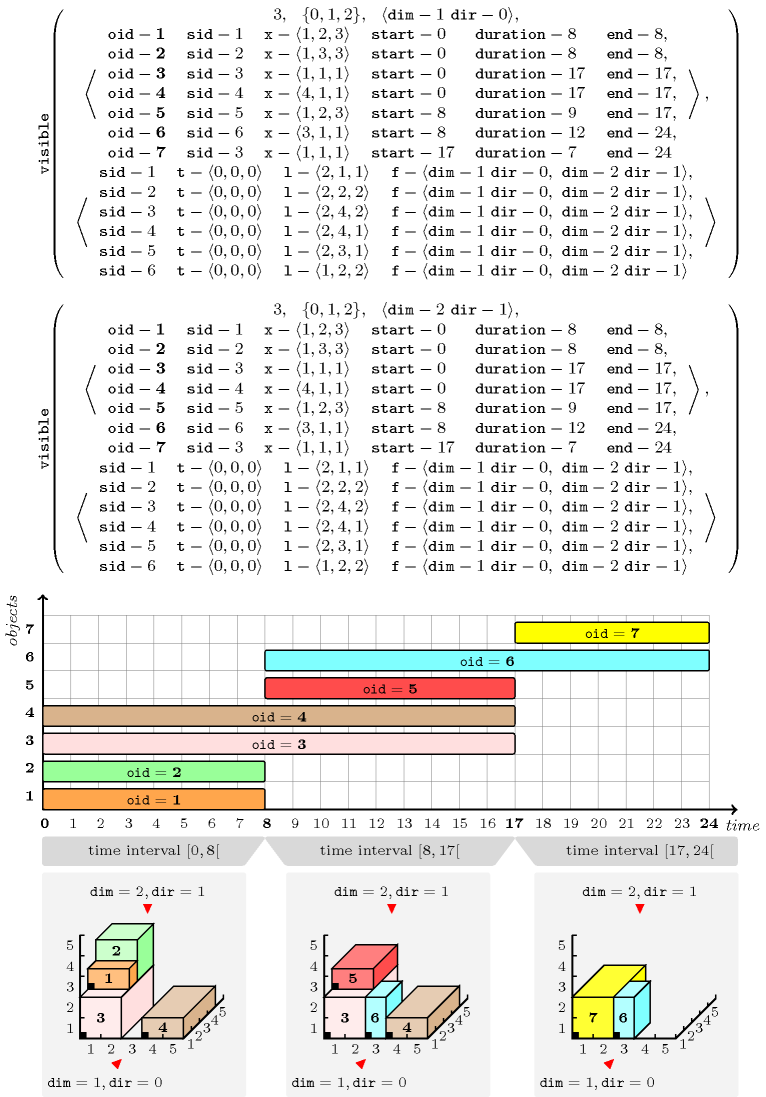• Figure 5.419.7 corresponds to a pallet loading problem where one has to place six objects on a pallet. Each object corresponds to a parallelepiped that has a bar code on one of its four sides (i.e., the sides that are different from the top and the bottom of the parallelepiped). If, for some reason, an object has no bar code then we simply remove it from the objects that will be passed to the $\mathrm{𝚟𝚒𝚜𝚒𝚋𝚕𝚎}$ constraint: this is for instance the case for the sixth object. In this context the constraint to enforce (beside the non-overlapping constraint between the parallelepipeds that are assigned to a same pallet) is the fact that the bar code of each object should be visible (i.e., visible from one of the four sides of the pallet). This is expressed by the $\mathrm{𝚟𝚒𝚜𝚒𝚋𝚕𝚎}$ constraint given in Part (F) of Figure 5.419.7.The $\mathrm{𝚟𝚒𝚜𝚒𝚋𝚕𝚎}$ constraint is a generalisation of the $\mathrm{𝚊𝚌𝚌𝚎𝚜𝚜𝚒𝚋𝚒𝚕𝚒𝚝𝚢}$ constraint initially introduced in the context of the $\mathrm{𝚍𝚒𝚏𝚏𝚗}$ constraint.